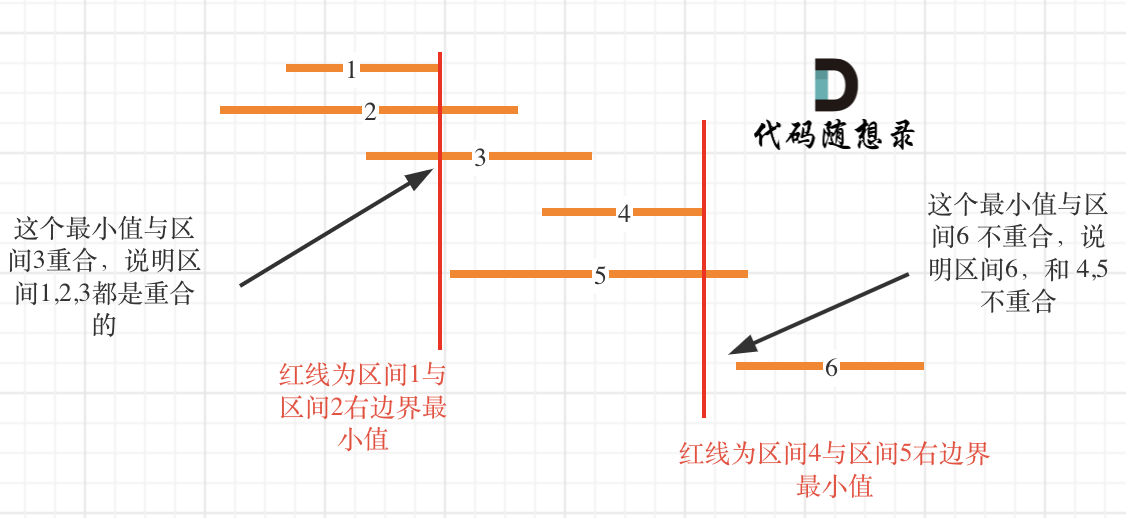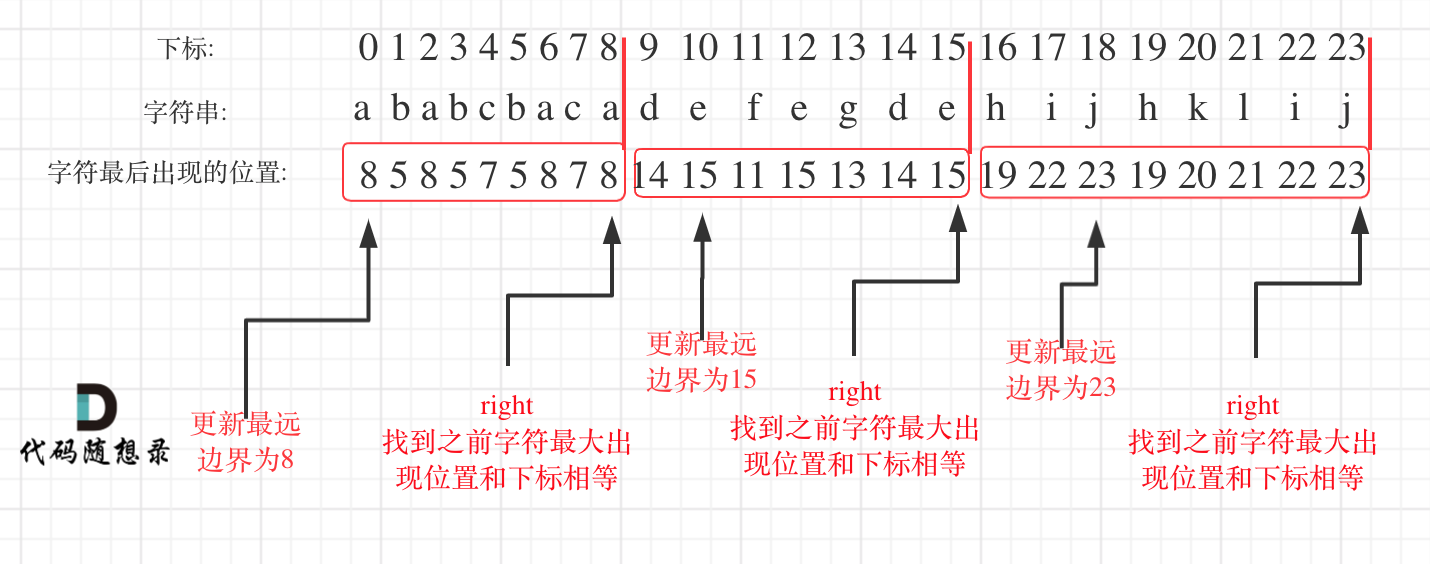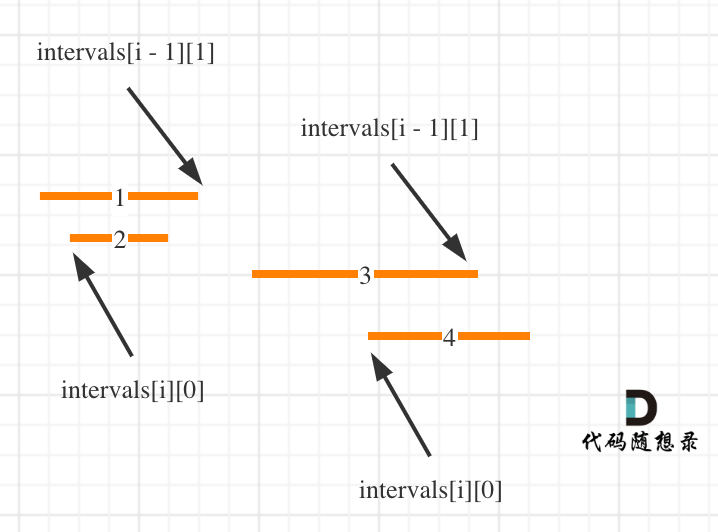## 【LeetCode题目详解】第八章 贪心算法 part05 435. 无重叠区间 763.划分字母区间 56. 合并区间 （day36补）

article2023/10/3 18:50:55/

# 一、力扣第435题：无重叠区间

## 题目：

```输入: intervals = [[1,2],[2,3],[3,4],[1,3]]

```

```输入: intervals = [ [1,2], [1,2], [1,2] ]

```

```输入: intervals = [ [1,2], [2,3] ]

```

• `1 <= intervals.length <= 105`
• `intervals[i].length == 2`
• `-5 * 104 <= starti < endi <= 5 * 104`

## 思路C++代码如下：

``````class Solution {
public:
// 按照区间右边界排序
static bool cmp (const vector<int>& a, const vector<int>& b) {
return a < b;
}
int eraseOverlapIntervals(vector<vector<int>>& intervals) {
if (intervals.size() == 0) return 0;
sort(intervals.begin(), intervals.end(), cmp);
int count = 1; // 记录非交叉区间的个数
int end = intervals; // 记录区间分割点
for (int i = 1; i < intervals.size(); i++) {
if (end <= intervals[i]) {
end = intervals[i];
count++;
}
}
return intervals.size() - count;
}
};
``````
• 时间复杂度：O(nlog n) ，有一个快排
• 空间复杂度：O(n)，有一个快排，最差情况(倒序)时，需要n次递归调用。因此确实需要O(n)的栈空间

## # 补充

### # 补充（1）

``````class Solution {
public:
static bool cmp (const vector<int>& a, const vector<int>& b) {
return a < b; // 改为左边界排序
}
int eraseOverlapIntervals(vector<vector<int>>& intervals) {
if (intervals.size() == 0) return 0;
sort(intervals.begin(), intervals.end(), cmp);
int count = 0; // 注意这里从0开始，因为是记录重叠区间
int end = intervals; // 记录区间分割点
for (int i = 1; i < intervals.size(); i++) {
if (intervals[i] >= end)  end = intervals[i]; // 无重叠的情况
else { // 重叠情况
end = min(end, intervals[i]);
count++;
}
}
return count;
}
};
``````

``````class Solution {
public:
static bool cmp (const vector<int>& a, const vector<int>& b) {
return a < b; // 改为左边界排序
}
int eraseOverlapIntervals(vector<vector<int>>& intervals) {
if (intervals.size() == 0) return 0;
sort(intervals.begin(), intervals.end(), cmp);
int count = 0; // 注意这里从0开始，因为是记录重叠区间
for (int i = 1; i < intervals.size(); i++) {
if (intervals[i] < intervals[i - 1]) { //重叠情况
intervals[i] = min(intervals[i - 1], intervals[i]);
count++;
}
}
return count;
}
};

``````

### # 补充（2）

(opens new window)非常像，弓箭的数量就相当于是非交叉区间的数量，只要把弓箭那道题目代码里射爆气球的判断条件加个等号（认为[0，1][1，2]不是相邻区间），然后用总区间数减去弓箭数量 就是要移除的区间数量了。

(opens new window)代码稍做修改，就可以AC本题。

``````class Solution {
public:
// 按照区间右边界排序
static bool cmp (const vector<int>& a, const vector<int>& b) {
return a < b; // 右边界排序
}
int eraseOverlapIntervals(vector<vector<int>>& intervals) {
if (intervals.size() == 0) return 0;
sort(intervals.begin(), intervals.end(), cmp);

int result = 1; // points 不为空至少需要一支箭
for (int i = 1; i < intervals.size(); i++) {
if (intervals[i] >= intervals[i - 1]) {
result++; // 需要一支箭
}
else {  // 气球i和气球i-1挨着
intervals[i] = min(intervals[i - 1], intervals[i]); // 更新重叠气球最小右边界
}
}
return intervals.size() - result;
}
};
``````

``````class Solution {
public:
// 按照区间左边界排序
static bool cmp (const vector<int>& a, const vector<int>& b) {
return a < b; // 左边界排序
}
int eraseOverlapIntervals(vector<vector<int>>& intervals) {
if (intervals.size() == 0) return 0;
sort(intervals.begin(), intervals.end(), cmp);

int result = 1; // points 不为空至少需要一支箭
for (int i = 1; i < intervals.size(); i++) {
if (intervals[i] >= intervals[i - 1]) {
result++; // 需要一支箭
}
else {  // 气球i和气球i-1挨着
intervals[i] = min(intervals[i - 1], intervals[i]); // 更新重叠气球最小右边界
}
}
return intervals.size() - result;
}
};

``````

# 二、力扣第763题：划分字母区间

## 题目：

```输入：s = "ababcbacadefegdehijhklij"

```输入：s = "eccbbbbdec"

```

• `1 <= s.length <= 500`
• `s` 仅由小写英文字母组成

## 思路

• 统计每一个字符最后出现的位置
• 从头遍历字符，并更新字符的最远出现下标，如果找到字符最远出现位置下标和当前下标相等了，则找到了分割点``````class Solution {
public:
vector<int> partitionLabels(string S) {
int hash = {0}; // i为字符，hash[i]为字符出现的最后位置
for (int i = 0; i < S.size(); i++) { // 统计每一个字符最后出现的位置
hash[S[i] - 'a'] = i;
}
vector<int> result;
int left = 0;
int right = 0;
for (int i = 0; i < S.size(); i++) {
right = max(right, hash[S[i] - 'a']); // 找到字符出现的最远边界
if (i == right) {
result.push_back(right - left + 1);
left = i + 1;
}
}
return result;
}
};
``````
• 时间复杂度：O(n)
• 空间复杂度：O(1)，使用的hash数组是固定大小

## # 补充

(opens new window)相同的思路。

(opens new window)题目里的输入)，将区间按左边界从小到大排序，找到边界将区间划分成组，互不重叠。找到的边界就是答案。

``````class Solution {
public:
static bool cmp(vector<int> &a, vector<int> &b) {
return a < b;
}
// 记录每个字母出现的区间
vector<vector<int>> countLabels(string s) {
vector<vector<int>> hash(26, vector<int>(2, INT_MIN));
vector<vector<int>> hash_filter;
for (int i = 0; i < s.size(); ++i) {
if (hash[s[i] - 'a'] == INT_MIN) {
hash[s[i] - 'a'] = i;
}
hash[s[i] - 'a'] = i;
}
// 去除字符串中未出现的字母所占用区间
for (int i = 0; i < hash.size(); ++i) {
if (hash[i] != INT_MIN) {
hash_filter.push_back(hash[i]);
}
}
return hash_filter;
}
vector<int> partitionLabels(string s) {
vector<int> res;
// 这一步得到的 hash 即为无重叠区间题意中的输入样例格式：区间列表
// 只不过现在我们要求的是区间分割点
vector<vector<int>> hash = countLabels(s);
// 按照左边界从小到大排序
sort(hash.begin(), hash.end(), cmp);
// 记录最大右边界
int rightBoard = hash;
int leftBoard = 0;
for (int i = 1; i < hash.size(); ++i) {
// 由于字符串一定能分割，因此,
// 一旦下一区间左边界大于当前右边界，即可认为出现分割点
if (hash[i] > rightBoard) {
res.push_back(rightBoard - leftBoard + 1);
leftBoard = hash[i];
}
rightBoard = max(rightBoard, hash[i]);
}
// 最右端
res.push_back(rightBoard - leftBoard + 1);
return res;
}
};
``````

# 三、力扣第56题：合并区间

## 题目：

```输入：intervals = [[1,3],[2,6],[8,10],[15,18]]

```

```输入：intervals = [[1,4],[4,5]]

• `1 <= intervals.length <= 104`
• `intervals[i].length == 2`
• `0 <= starti <= endi <= 104`

## 思路

(opens new window) 和 435. 无重叠区间

(opens new window) 都是一个套路。C++代码如下：

``````class Solution {
public:
vector<vector<int>> merge(vector<vector<int>>& intervals) {
vector<vector<int>> result;
if (intervals.size() == 0) return result; // 区间集合为空直接返回
// 排序的参数使用了lambda表达式
sort(intervals.begin(), intervals.end(), [](const vector<int>& a, const vector<int>& b){return a < b;});

// 第一个区间就可以放进结果集里，后面如果重叠，在result上直接合并
result.push_back(intervals);

for (int i = 1; i < intervals.size(); i++) {
if (result.back() >= intervals[i]) { // 发现重叠区间
// 合并区间，只更新右边界就好，因为result.back()的左边界一定是最小值，因为我们按照左边界排序的
result.back() = max(result.back(), intervals[i]);
} else {
result.push_back(intervals[i]); // 区间不重叠
}
}
return result;
}
};
``````
• 时间复杂度: O(nlogn)
• 空间复杂度: O(logn)，排序需要的空间开销

day36补

### 计算机网络-笔记-第三章-数据链路层

&#x1f338;章节汇总 一、第一章——计算机网络概述 二、第二章——物理层 三、第三章——数据链路层 四、第四章——网络层 五、第五章——运输层 六、第六章——应用层 目录 三、第三章——数据链路层 1、数据链路层概述&#xff08;帧&#xff09; &#xff08;1&…

### Spring5学习笔记—Spring事务处理

✅作者简介&#xff1a;大家好&#xff0c;我是Leo&#xff0c;热爱Java后端开发者&#xff0c;一个想要与大家共同进步的男人&#x1f609;&#x1f609; &#x1f34e;个人主页&#xff1a;Leo的博客 &#x1f49e;当前专栏&#xff1a; Spring专栏 ✨特色专栏&#xff1a; M…

### go Session的实现（一）

〇、前言 众所周知&#xff0c;http协议是无状态的&#xff0c;这对于服务器确认是哪一个客户端在发请求是不可能的&#xff0c;因此为了能确认到&#xff0c;通常方法是让客户端发送请求时带上身份信息。容易想到的方法就是客户端在提交信息时&#xff0c;带上自己的账户和密…